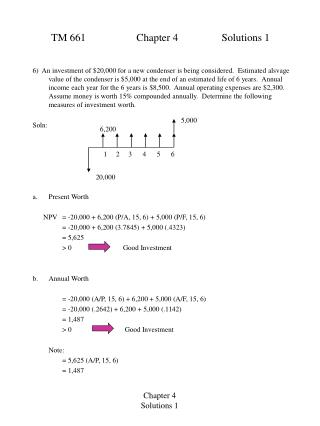DownloadDownload PresentationTM 661 Chapter 4 Solutions 1

# TM 661 Chapter 4 Solutions 1

Télécharger la présentation## TM 661 Chapter 4 Solutions 1

- - - - - - - - - - - - - - - - - - - - - - - - - - - E N D - - - - - - - - - - - - - - - - - - - - - - - - - - -
##### Presentation Transcript

1. 5,000 6,200 1 2 3 4 5 6 20,000 TM 661 Chapter 4 Solutions 1 6) An investment of \$20,000 for a new condenser is being considered. Estimated alsvage value of the condenser is \$5,000 at the end of an estimated life of 6 years. Annual income each year for the 6 years is \$8,500. Annual operating expenses are \$2,300. Assume money is worth 15% compounded annually. Determine the following measures of investment worth. Soln: a. Present Worth NPV = -20,000 + 6,200 (P/A, 15, 6) + 5,000 (P/F, 15, 6) = -20,000 + 6,200 (3.7845) + 5,000 (.4323) = 5,625 > 0 Good Investment b. Annual Worth = -20,000 (A/P, 15, 6) + 6,200 + 5,000 (A/F, 15, 6) = -20,000 (.2642) + 6,200 + 5,000 (.1142) = 1,487 > 0 Good Investment Note: = 5,625 (A/P, 15, 6) = 1,487 Chapter 4 Solutions 1

2. 5,000 6,200 1 2 3 4 5 6 20,000 TM 661 Chapter 4 Solutions 1 6) Cont. Soln: e. Internal Rate of Return Find i such that PWR(i) = PWC(i) 20,000 = 6,200 (P/A, i, 6) + 5,000 (P/F, i, 6) IRR = 24% e. External Rate of Return Find I such that PWR(15%) = PWC(i) 20,000 = 6,200 (P/A, 15, 6) + 5,000 (P/F, 15, 6) Note that this equation is not a function of i. Therefore, we can not use it. Instead, let us equate the Future worth of Costs with the Future worth of Revenues. 20,000(1+i)6 = 6,200 (F/A, 15, 6) + 5,000 Chapter 4 Solutions 2

3. TM 661 Chapter 4 Solutions 1 6) Cont. 20,000(1+i)6 = 6,200 (F/A, 15, 6) + 5,000 at i = 0.20 20,000 (1.2)6 = 6,200 (8.7537) + 5,000 59,720 = 65,838 at i = 0.22 20,000 (1.22)6 = 65,838 ERR = 22% (Note: Text gives ERR = 20%. Let me know if you see an error I’ve made.) f. Savings Investment Ratio SIR = PWR(15%) / PWC(15%) = 25,625 / 20,000 = 1.28 Chapter 4 Solutions 3

4. 5 10 . . . 50 250 250 2,000 TM 661 Chapter 4 Solutions 1 16) A flood control project has a construction cost of \$2,000,000, an annual maintenance cost of \$50,000, and a major repair at 5-year intervals of \$250,000. If the interest rate is 8% annually, determine the total capitalized cost required to fund the project. Soln: Find the equivalent annual maintenance. A = 50 + 250 (A/F, 8, 5) = 50 + 250 (.1705) = 92.625 Pc = P + A/i = 2,000 + 92.625/.08 = 3,157.812 Total capitalized cost for the project is \$3,157,812 Chapter 4 Solutions 4